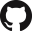# 50.034 Introduction to Probability and Statistics¶

No. of Credits: 12 Subject Credits

Pre-requisites: None

## Course Description¶

This course is an introduction to statistics and probability covering essential topics. Sample space, discrete and continuous random variables, probability distributions, joint and conditional distributions, expectation, transformation of random variables, limit theorems, estimation theory, hypothesis testing, confidence interval, statistical tests, and regression.

## Learning Objectives¶

• Apply key concepts of probability, including discrete and continuous random variables, probability distributions, conditioning, independence, expectations, and moments.
• Define and explain the different statistical distributions (e.g., normal, log-normal, Poisson, Weibull) and the typical phenomena that each distribution often describes.
• Apply the basic rules and theorems in probability including Bayes’s theorem and the Central Limit Theorem (CLT).
• Define and demonstrate the concepts of estimation and properties of estimators.
• Apply the concepts of interval estimation and confidence intervals.
• Apply the concepts of hypothesis testing and p-value.
• Apply the method of least squares to estimate the parameters in a regression model.
• Use standard software (e.g., Matlab) to facilitate statistical analysis.

## Measurable Outcomes¶

• Evaluate the probabilities and conditional probabilities.
• Evaluate expectations and conditional expectations of random variables.
• Approximate the distribution of sum of random variables using CLT.
• Construct point estimators using the method of maximum likelihood.
• Calculate the number of samples needed to construct confidence levels on the mean and variance of a normal distribution.
• Design hypothesis tests for a given set of data and select the appropriate thresholds for the tests.
• Use linear regression analysis to develop an empirical model of experimental data.
• Create computer programs to facilitate the analysis and representation of data.

## Course Notes¶

This set of course notes was graciously shared by Koh Jing Yu, updated as of 11 April 2018.